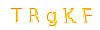## Java 8 flatMap example

In Java 8, Stream can hold different data types, for examples:

```Stream<String[]>
Stream<Set<String>>
Stream<List<String>>
Stream<List<Object>>
```

But, the Stream operations (filter, sum, distinct…) and collectors do not support it, so, we need flatMap() to do the following conversion :

```Stream<String[]>		-> flatMap ->	Stream<String>
Stream<Set<String>>	-> flatMap ->	Stream<String>
Stream<List<String>>	-> flatMap ->	Stream<String>
Stream<List<Object>>	-> flatMap ->	Stream<Object>
```

How flatMap() works :

```{ {1,2}, {3,4}, {5,6} } -> flatMap -> {1,2,3,4,5,6}
{ {'a','b'}, {'c','d'}, {'e','f'} } -> flatMap -> {'a','b','c','d','e','f'}
```

## 1. Stream + String[] + flatMap

1.1 The below example will print an empty result, because filter() has no idea how to filter a stream of String[].

TestExample1.java
```package com.mkyong.java8;
import java.util.Arrays;
import java.util.stream.Stream;
public class TestExample1 {
public static void main(String[] args) {
String[][] data = new String[][]{{"a", "b"}, {"c", "d"}, {"e", "f"}};
//Stream<String[]>
Stream<String[]> temp = Arrays.stream(data);
//filter a stream of string[], and return a string[]?
Stream<String[]> stream = temp.filter(x -> "a".equals(x.toString()));
stream.forEach(System.out::println);
```

Output

```//empty...
```

1.2 In above example, we should use flatMap() to convert Stream<String[]> to Stream<String>.

TestExample1.java
```package com.mkyong.java8;
import java.util.Arrays;
import java.util.stream.Stream;
public class TestExample1 {
public static void main(String[] args) {
String[][] data = new String[][]{{"a", "b"}, {"c", "d"}, {"e", "f"}};
//Stream<String[]>
Stream<String[]> temp = Arrays.stream(data);
//Stream<String>, GOOD!
Stream<String> stringStream = temp.flatMap(x -> Arrays.stream(x));
Stream<String> stream = stringStream.filter(x -> "a".equals(x.toString()));
stream.forEach(System.out::println);
/*Stream<String> stream = Arrays.stream(data)
.flatMap(x -> Arrays.stream(x))
.filter(x -> "a".equals(x.toString()));*/
```

Output

## 2. Stream + Set + flatMap

2.1 A student POJO.

Student.java
```package com.mkyong.java8;
import java.util.HashSet;
import java.util.Set;
public class Student {
private String name;
private Set<String> book;
if (this.book == null) {
this.book = new HashSet<>();
//getters and setters
```

2.2 flatMap() and Set example.

TestExample2.java
```package com.mkyong.java8;
import java.util.ArrayList;
import java.util.List;
import java.util.stream.Collectors;
public class TestExample2 {
public static void main(String[] args) {
Student obj1 = new Student();
obj1.setName("mkyong");
Student obj2 = new Student();
obj2.setName("zilap");
List<Student> list = new ArrayList<>();
List<String> collect =
list.stream()
.map(x -> x.getBook())      //Stream<Set<String>>
.flatMap(x -> x.stream())   //Stream<String>
.distinct()
.collect(Collectors.toList());
collect.forEach(x -> System.out.println(x));
```

Output

```Spring Boot in Action
Effective Java (2nd Edition)
Java 8 in Action
Learning Python, 5th Edition
```

Try comments the flatMap(x -> x.stream()) the Collectors.toList() will prompts a compiler error, because it has no idea how to collect a stream of Set object.

## 3. Stream + Primitive + flatMapToInt

3.1 For primitive type, you can use flatMapToInt.

TestExample3.java
```package com.mkyong.java8;
import java.util.Arrays;
import java.util.stream.IntStream;
import java.util.stream.Stream;
public class TestExample3 {
public static void main(String[] args) {
int[] intArray = {1, 2, 3, 4, 5, 6};
//1. Stream<int[]>
Stream<int[]> streamArray = Stream.of(intArray);
//2. Stream<int[]> -> flatMap -> IntStream
IntStream intStream = streamArray.flatMapToInt(x -> Arrays.stream(x));
intStream.forEach(x -> System.out.println(x));
```

Output

## References

评论 ( What Do You Think )
 名称 邮箱 网址 评论 验证•微信公众号
•我的微信

1、一号门博客CMS,由Python, MySQL, Nginx, Wsgi 强力驱动

2、部分文章或者资源来源于互联网, 有时候很难判断是否侵权, 若有侵权, 请联系邮箱：summer@yihaomen.com, 同时欢迎大家注册用户，主动发布无版权争议的 文章/资源.